Next: Flux conversions Up: FEASIBILITY: THE WIDE FIELD Previous: Introduction

# Minimum detectable count rates

The minimum detectable count rate for the WFC depends mainly on the background count rate, and the effective size of an unresolved source. The minimum detectable count rate,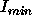, can be estimated from the following formula (e.g., de Korte et al., 1981, Space Science Reviews, 30, p. 495-511):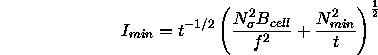where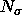is the required significance threshold (i.e., signal-to-noise),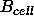is the background count rate in the ``detection cell'', f is the fraction of the total source count included in the ``detection cell'',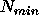is the minimum number of photons required to constitute a detection and t is the integration time.

The ``detection cell'' is the region of the image used to detect the source. For the WFC, assuming an on-axis source, an appropriate cell area depends on the filter used, since the point response function is energy-dependent. For the S1, S2 and P1 filters the appropriate radius enclosing 70% of the total count (i.e.,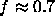) is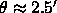, whilst for P2,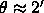. The corresponding cell areas,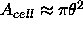, are thus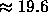arcminand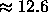arcminrespectively. The count rates in the background cell are then calculated as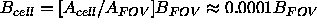, where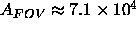arcminis the area of the whole field of view and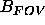it the total background count rate of the whole field.

The minimum detectable count rateas a function of t is shown graphically in Figure 12.1for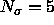, f=0.7,. Three curves are shown, the two labelled `LOW' and `HIGH' are to be used for the S1,S2, P1 filters and are plotted for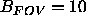and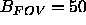counts s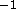, i.e. for the typical range of background rates expected. The low background rates are only achieved for restricted time intervals, guest investigators should therefore assume the `HIGH' curve when proposing. The curve labelled P2 is to be used for observations with the P2 filter and assumes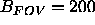counts s, i.e. the night-time background rate expected in this filter (see § 7.8.4).Next: Flux conversions Up: FEASIBILITY: THE WIDE FIELD Previous: Introduction

Michael Arida
Tue Jun 11 16:18:41 EDT 1996